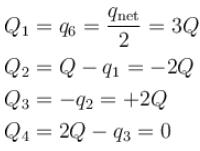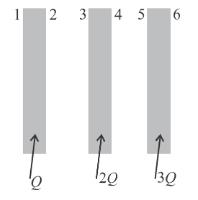Courses

# Electrostatics NAT Level - 2

## 10 Questions MCQ Test Topic wise Tests for IIT JAM Physics | Electrostatics NAT Level - 2

Description
This mock test of Electrostatics NAT Level - 2 for Physics helps you for every Physics entrance exam. This contains 10 Multiple Choice Questions for Physics Electrostatics NAT Level - 2 (mcq) to study with solutions a complete question bank. The solved questions answers in this Electrostatics NAT Level - 2 quiz give you a good mix of easy questions and tough questions. Physics students definitely take this Electrostatics NAT Level - 2 exercise for a better result in the exam. You can find other Electrostatics NAT Level - 2 extra questions, long questions & short questions for Physics on EduRev as well by searching above.
*Answer can only contain numeric values
QUESTION: 1

### Figure shows a solid hemisphere with a charge of 5nC  distributed uniformly throughout its volume. The hemisphere lies on a plane and point P is located on the plane, along a radial line from the centre of curvature at distance 15cm. The electric potential at point P (in volts) due to the hemisphere, is___.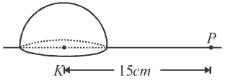Solution:

By argument of symmetry, it will be half of the potential produced by the full sphere

∵ – Charge on hemisphere = Q, so charge on sphere = 2Q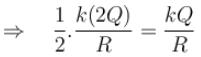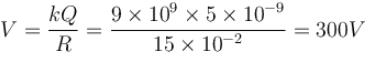*Answer can only contain numeric values
QUESTION: 2

### A simple pendulum with a bob of mass m = 1kg, charge q = 5μC and string length l = 1m is given a horizontal velocity u in a uniform electric field E = 2 × 106 V/m  at its bottom most point A, as shown in figure. It is given that the speed u is such that the particle leaves the circle at point C. Find the speed u.  (Take g = 10m/s2)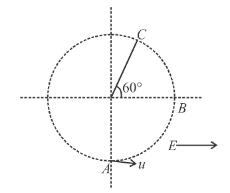Solution:

From work-energy theorem,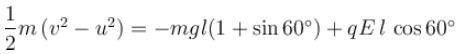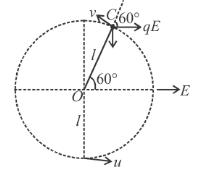Substituting the values, we get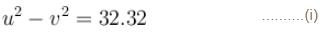Further, at C tension in the string is zero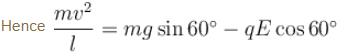or  v2 = 3.66 ......(ii)
From Eqs. (i) and (ii), we get

u = 6m/s

*Answer can only contain numeric values
QUESTION: 3

### A position charge +Q is fixed at a point A. Another positively charged. particle of mass m and charge +q is projected from a point B with velocity u as shown in the figure. The point B is large distance from A and at distance d from the line AC. The initial velocity is parallel to the line AC. The point C is at very large distance from A. Find the minimum distance (in meter) of +q from +Q during the motion. Take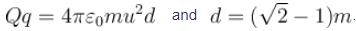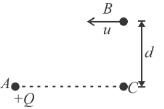Solution:

The path of the particle will be as shown in the figure. At the point of minimum distance (D) the velocity of the particle will be to its position vector w.r.t +Q.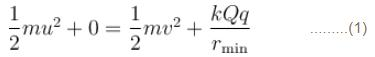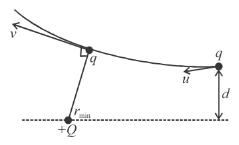∵ Torque on q about Q is zero hence angular momentum about Q will be conserved
⇒  mvrmin = mud      ........(2)
by (2) in (1)∵   distance cannot be negative
∴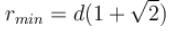*Answer can only contain numeric values
QUESTION: 4

The electric potential varies in space according to the relation V = 3x + 4y. A particle of mass 10kg starts from rest from point (2,3.2)m under the influence of this field. Find the velocity of the particle in units of 10–3m/s, when it crosses the x-axis. The charge on the particle is 1μC. Assume V(x,y) are in SI units.

Solution: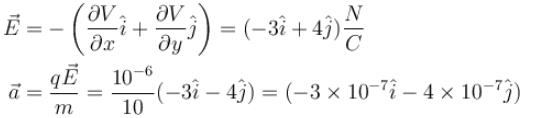When particle crosses x-axis, y = 0

Initial y-coordinate was 3.2 m and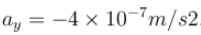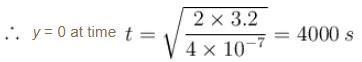At this instant x-coordinate will be,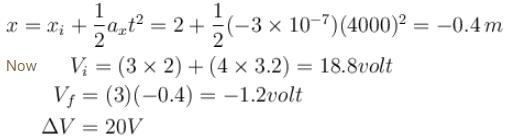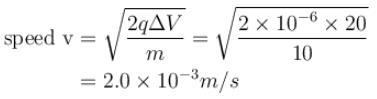*Answer can only contain numeric values
QUESTION: 5

Consider a cube of side a = 0.1m  placed such that its six faces are given by equations x = 0, x = + a, y = 0, y = +a, z = 0 and z = +a, placed in electric field given by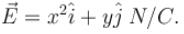Find the electric flux crossing out of the cube in the unit of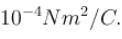Solution: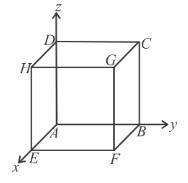Flux through ABCD.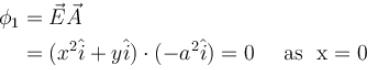Flux through BCGF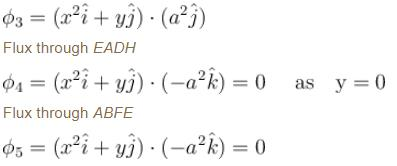Flux through CDHG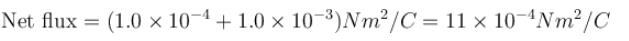*Answer can only contain numeric values
QUESTION: 6

A solid sphere of radius R has a cavity of radius R/2 The solid part has a uniform charge density ρ and cavity has no charge. The electric potential at point A is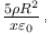then value of x is

Solution:

Assume a solid sphere without cavity.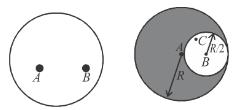Potential at A due to this solid sphere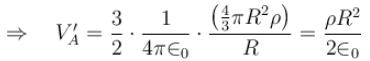Electric field at C due to this solid sphere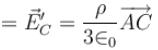Now consider the cavity filled with negative charge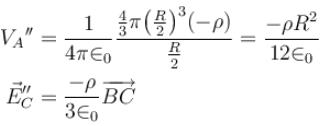Now net values for the solid sphere with the cavity can be given by superposition of the above two cases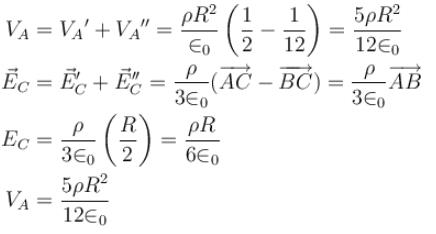*Answer can only contain numeric values
QUESTION: 7

A graph of the x component of the electric field as a function of x in a region of space is shown. The y and z components of the electric field are zero in this region. If the electric potential is 10V at the origin, then potential (in volts) at x = 2.0m is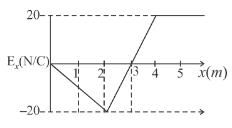Solution: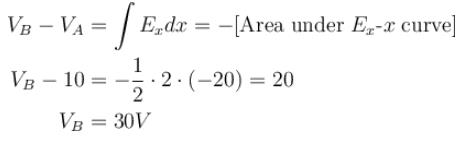*Answer can only contain numeric values
QUESTION: 8

A dipole of dipole moment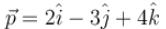is placed at point A(2, -3, 1). The electric potential due to this dipole at the point B(4, -1, 0) is equal to n × 109 volts. Find the value of n.

Solution: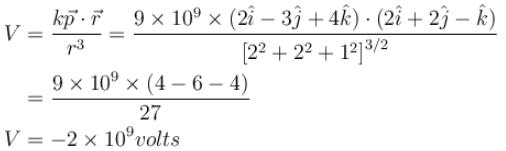*Answer can only contain numeric values
QUESTION: 9

A 4.00 kg block carrying a charge Q = 50.0μC is connected to a spring for which k = 100N/m. The block lies on a friction less horizontal track, and the system is immersed in a uniform electric field of magnitude E = 5.00 × 105 V/m, directed as shown in figure. If the block is released from rest when the spring is unscratched (at x = 0). By what maximum amount does the spring expand. (Answer in meters)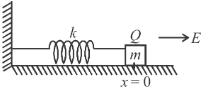Solution:

By comparing this problem with spring-block system problem suspended vertically.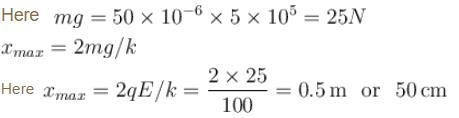*Answer can only contain numeric values
QUESTION: 10

Three identical, conducting plane parallel plates, each of area A are held with equal separation d between successive surfaces. Charges Q, 2Q, and 3Q are placed on them. Neglecting edge effects. The sum of charges on the six surfaces is given by nQ. Find the value of n.

Solution: